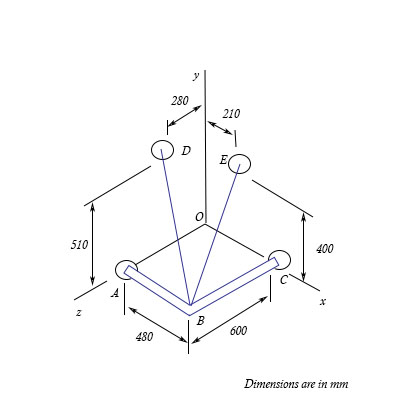# A frame ABC is supported in part by cable DBE that passes through a frictionless ring at B....

## Question:

A frame {eq}ABC {/eq} is supported in part by cable {eq}DBE {/eq} that passes through a frictionless ring at {eq}B {/eq}. Determine the magnitude and direction of resultant forces exerted by the cable at {eq}B {/eq} knowing that the tension in the cable is {eq}435 N {/eq}. Also, determine the components of the force exerted by the cable on the supports at {eq}D. {/eq} ( Round the resultant to the nearest number, and the directions to a single decimal place).## Resultant force

A single force that is able to replace all other forces that are acting on a body is termed as a resultant force. The resultant force is also exerted the same effect on the body as that of the system of forces.

Become a Study.com member to unlock this answer! Create your account

Given data:

• The tension in the cord of BD and BE is: {eq}T = 435\;{\rm{N}} {/eq}.
• The position vector of B is: {eq}{\vec r_B} = \left( {480\hat i...Resultant Force: Definition & Formula

from

Chapter 18 / Lesson 5
42K

In lots of situations, more than one force may act on an object. In this lesson, learn how to find the resultant force of two forces on a single object and how to use this to determine how the object will move.